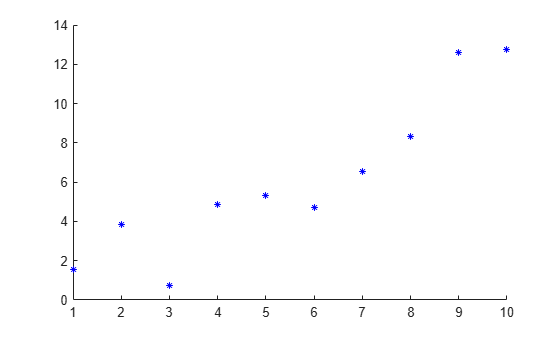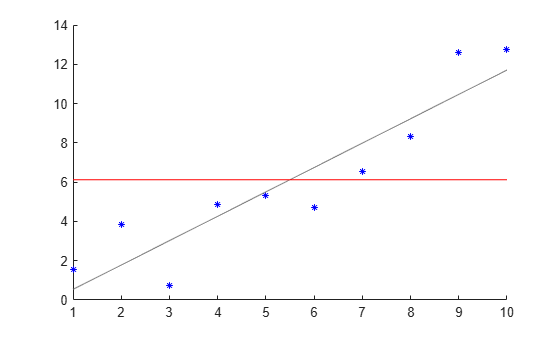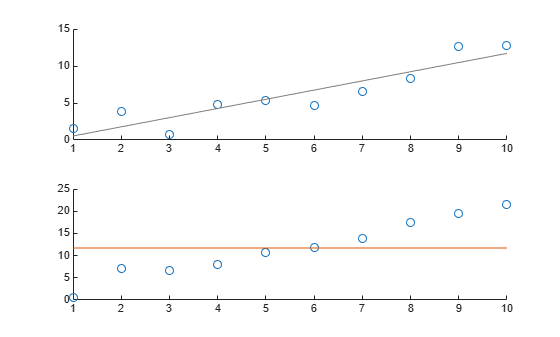refline

Description

refline(m,b) adds a reference line with slope m and intercept b to the current axes.

example

refline(coeffs) adds the line defined by the elements of the vector coeffs to the figure.

example

refline with no input arguments is equivalent to lsline.

example

refline(ax,___) adds a reference line to the plot in the axis specified by ax, using any of the input arguments in the previous syntaxes.

example

hline = refline(___) returns the reference line object hline using any of the input arguments in the previous syntaxes. Use hline to modify properties of a specific reference line after you create it. For a list of properties, see Line Properties.

Examples

collapse all

Generate sample data for an independent variable x and a dependent variable y .

x = 1:10;
y = x + randn(1,10);

Create a scatter plot of x and y .

scatter(x,y,25,'b','*')Superimpose a least-squares line on the scatter plot.

reflineAdd a reference line at the mean of the scatter plot.

mu = mean(y);
hline = refline([0 mu]);
hline.Color = 'r';The red line is the reference line at the mean of the data.

Define the x-variable and two different y-variables to use for the plots.

rng default  % For reproducibility
x = 1:10;
y1 = x + randn(1,10);
y2 = 2*x + randn(1,10);

Define ax1 as the top half of the figure, and ax2 as the bottom half of the figure. Create the first scatter plot on the top axis using y1, and the second scatter plot on the bottom axis using y2.

figure
ax1 = subplot(2,1,1);
ax2 = subplot(2,1,2);

scatter(ax1,x,y1)
scatter(ax2,x,y2)Superimpose a least-squares line on the top plot, and a reference line at the mean of the y2 values in the bottom plot.

lsline(ax1) % This is equivalent to refline(ax1)

mu = mean(y2);
refline(ax2,[0 mu])Input Arguments

collapse all

Slope of the reference line, specified as a numeric scalar. The function uses m to define the line

y = m*x + b.

Example: refline(-1,1)

Data Types: single | double

Intercept of the reference line, specified as a numeric scalar. The function uses b to define the line

y = m*x + b.

Example: refline(2,-10)

Data Types: single | double

Linear coefficients, specified as a length-two numeric vector. coeffs contains the coefficients of a line defined as

y = coeffs(1)*x + coeffs(2).

Example: refline([-1,2])

Data Types: single | double

Target axes, specified as an axes object. If you do not specify the axes and if the current axes are Cartesian axes, then the refline function uses the current axes.

Output Arguments

collapse all

One or more reference line objects, returned as a scalar or a vector. These objects are unique identifiers, which you can use to query and modify properties of a specific reference line. For a list of properties, see Line Properties.